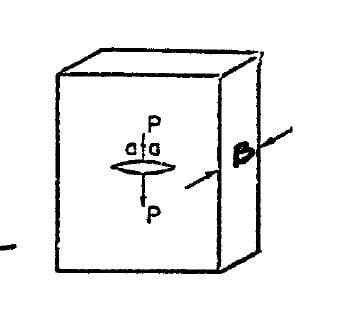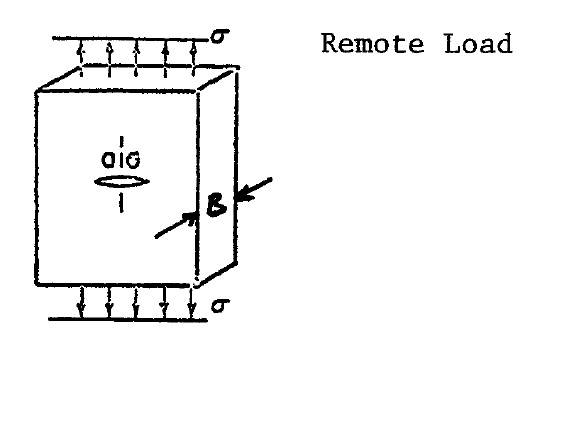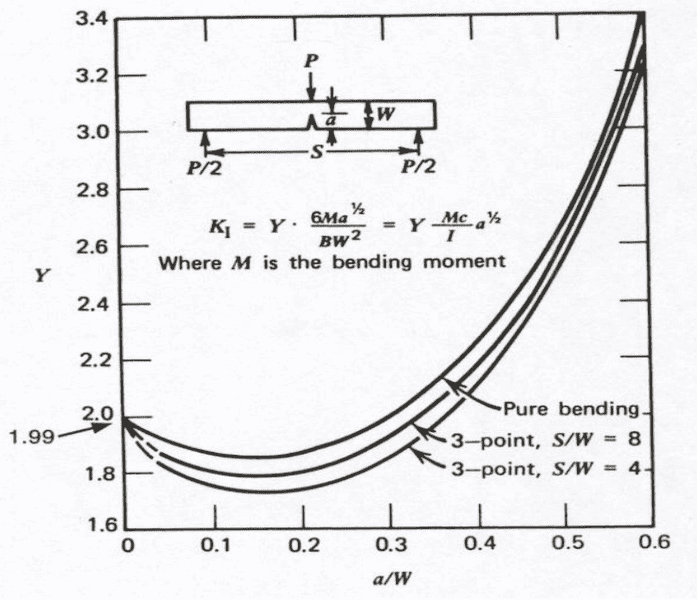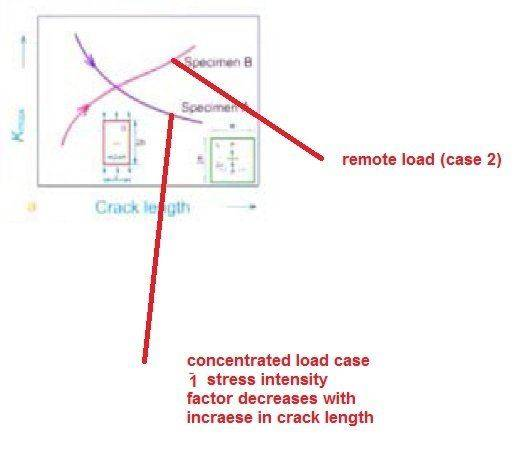# Fracture mechanics - understanding required

I had posted this in the General Engineering forum but perhaps it is a better question for mechanical engineering forum:

1) Consider a centre crack specimen subjected to a wedge load a shown in the figure:
https://www.physicsforums.com/attachments/centre_crack_wedge_load-jpg.112584/ [Broken]

In such a case the stress intensity factor DECREASES with the INCREASE in crack length

2) Now, consider a centre crack specimen subjected to a far field stress as shown below

https://www.physicsforums.com/attachments/centre_crack_far_field_stress-jpg.112585/ [Broken]

Here the stress intensity factor INCREASES with increase in crack length

Now, my question is,

Case 2 is intuitively appealing / understandable since with increase in crack length we move towards the fracture toughness of the material thus stress intensity factor increases with increasing crack length

I'm not able to intuitively understand case 1. With increase in crack length the stress intensity factor is decreasing and in fact with increasing crack length we are moving away from fracture toughness of the material?

Can anyone help, please?

Last edited by a moderator:

## Answers and Replies

Mapes
Science Advisor
Homework Helper
Gold Member
Your images aren't appearing for me---maybe others are having the same problem?

1) Below is the image corresponding to point 1)2) And below is the image corresponding to point 2Mapes
Science Advisor
Homework Helper
Gold Member
TLDR: In Case 2, the point load is distributed over a larger area when the crack is longer, weakening the load's influence. The same can't be said for Case 1.

Here's how I reason this out, although you may not find it satisfying:

First, let's note that you're focusing on the ##\frac{1}{\sqrt{\pi a}}## and ##\sqrt{\pi a}## factors, not on the geometric factor sometimes denoted by ##Y## that also appears in the stress intensity factor that distinguishes the infinite-width case from the finite-width case.

In Case 2, there's essentially no strain energy stored in the specimen directly above and below the crack, because the crack surfaces are stress-free away from the tip. Therefore, if the crack grows slightly, it will relieve the strain energy in the material above and below the new length segments. Reducing energy is what drives spontaneous processes, so the stress intensity factor increases. In addition, for finite plates, the geometric factor increases with increasing crack length because the load is being borne by a smaller amount of remaining material.

In Case 1, nearly all of the strain energy is stored directly above and below the crack, because the concentrated (or point) load is applied in this region. The regions to the left and right are largely unaffected. But for a larger crack, less energy ends up stored above and below the larger crack because the point load is distributed over a larger area, so the stress intensity factor for an infinite-width plate is lower, (i.e., the difference between Case 2 and 1 is ##K=\sigma\sqrt{\pi a}## vs. ##K=P/\sqrt{\pi a}##, respectively, for the infinite-width plate). With a finite-width plate, the crack may be so large the stress in the left and right regions is no longer negligible—after all, these regions must counteract the point load. This transition corresponds to a sharp increase in the geometric factor such that the stress intensity factor (for the finite plate) increases again with increasing crack length (see Fig. 8 here, noting that although the caption says "Stress-intensity factors", the y axis is labeled as the geometric factor).

So that kind of makes sense to me. However, I find it harder to explain the case of bending of a beam with a crack:I'd have to think about this one for a while before I can see why the stress intensity initially decreases. Here, it appears that ##Y## must decrease with increasing crack length. Could have something to do with the fact that the normal stress in a beam under bending transitions linearly from tensile to compressive as you move from the bottom of the beam to the top. I welcome your thoughts.

Last edited:
Hi,

In Case 2, there's essentially no strain energy stored in the specimen directly above and below the crack, because the crack surfaces are stress-free away from the tip.

Do you mean that there is more energy availability above and below the crack so that the crack gets the requisite energy to grow?

In Case 1, nearly all of the strain energy is stored directly above and below the crack, because the concentrated (or point) load is applied in this region

Do you mean that in this case there is less energy availability for the crack to grow?

IF the answer to the above questions is: YES, we still do not know WHY the stress intensity factor decreases in case 1. IF there is less energy availability (in case 1), the SIF in case 1 should be just less than that of case 2 but NOT DECREASE with increase in crack length.Last edited:
256bits
Gold Member
we still do not know WHY the stress intensity factor decreases in case 1
You should think of it , K, as the comparison of stress at the crack with that of the nominal stress produced by the applied load, and not as the increase in absolute total stress at the crack.

Draw some stress lines for both cases - field stress and wedge stress. If that helps.
For the wedge stress, note that some of the stress is taken up by compression of material above the crack, but that results in tension in the remaining material outside the crack. How does that compression translate to tension in and around the crack tip and remaining material outside the crack to the edge in the plane of the crack. What is the comparison of the stress in the remaining material to that at the crack tip?

A horrendously horrendous subject, and I would be pleased if that helps, but not sure if it will.

Anyways, I sat on the post for a while as my mind wandered over to how this thing about cracks and stress is similar, at least in principle, if not application, to flanges, gaskets and cylinder heads, bolted joints.

Mapes
Science Advisor
Homework Helper
Gold Member
IF there is less energy availability (in case 1), the SIF in case 1 should be just less than that of case 2 but NOT DECREASE with increase in crack length.View attachment 112805

The way I interpret it is: For increasing crack length, progressively less strain energy would be relieved in a infinite-width plate upon infinitesimal crack growth in Case 1.

256 bits,

For the wedge stress, note that some of the stress is taken up by compression of material above the crack, but that results in tension in the remaining material outside the crack. How does that compression translate to tension in and around the crack tip and remaining material outside the crack to the edge in the plane of the crack.

Do you mean that the stress intensity factor decreases in the case of the wedge loading, because part of the material (around the crack tip) is in compression and this not the case in the remote loading?

What is the comparison of the stress in the remaining material to that at the crack tip?

The stress intensity factors / stress field expressions derived based on the theory of linear elastic fracture mechanics are valid only in the region around the crack tip. Thus, I did not present the comparison in the remaining material.

PhanthomJay
Science Advisor
Homework Helper
Gold Member
Kaja....,

So Case 2 shows increase while case 1 shows decrease. This is a common problem when residual stress (under a point load P) will decrease when a far field stress is applied. The residual stress intensity is reduced as the crack grows stably then will stop growing as long as stress is subcritical. If the applied stress is held and moisture added (for most brittle materials), however, the stress intensity and crack will increase in depth and eventually fail

So Case 2 shows increase while case 1 shows decrease. This is a common problem when residual stress (under a point load P) will decrease when a far field stress is applied. The residual stress intensity is reduced as the crack grows stably then will stop growing as long as stress is subcritical. If the applied stress is held and moisture added (for most brittle materials), however, the stress intensity and crack will increase in depth and eventually fail

1) Sorry, but you were not clear to me
2) Why do you use the term "residual"
3) As you case 2 is remote loading and case 1 is point load

You say:

residual stress (under a point load P) will decrease when a far field stress is applied

The point load and the far filed stress are not in the same problem (case). Each is a separate case

4) I am asking what is the physical reason that why is the stress intensity factor decreasing in case 1 (only the point load acting)

5) In case 2, the stress intensity factor increases as crack length increases. This is intuitively fine to imagine

6)But in case 1, the stress intensity factor is DECREASING with increase in crack length. This is not intuitive

Thanks a lot,
Kajal

PhanthomJay
Science Advisor
Homework Helper
Gold Member
1) Sorry, but you were not clear to me
2) Why do you use the term "residual"
3) As you case 2 is remote loading and case 1 is point load

You say:

The point load and the far filed stress are not in the same problem (case). Each is a separate case

4) I am asking what is the physical reason that why is the stress intensity factor decreasing in case 1 (only the point load acting)

5) In case 2, the stress intensity factor increases as crack length increases. This is intuitively fine to imagine

6)But in case 1, the stress intensity factor is DECREASING with increase in crack length. This is not intuitive

Thanks a lot,
Kajal
I think Mapes and 256bits gave good responses to (6) to what 256bits correctly calls a "horrendously horrendous subject". (!!).

I mentioned residual stress because I find it hard to imagine a continuously applied 'point wedge load' ; rather, I'm envisioning the case where say a diamond was pressed with a force against say the glass, causing the crack, and then released, in which case there is a 'residual' stress at the crack, and the residual stress intensity will decrease as the crack grows (greater area) and then stops growing and the glass is weakened but will not fail as long as the stress is subcritical, where the critical stress intensity factor, unlike the stress intensity factor, is a fixed property of the material only. You should note that the stress intensity equations given by Mapes for the far field stress and then the 'point' load, with the former showing the (a^1/2 ) in the numerator and the latter having the (a^1/2) in the denominator, with the respective increase or decrease in stress intensity, have consistent units, provide that what you call a 'point' load is actually a 'line' load in units of force per unit length. For an idealized point load in force units, the (a^1/2) term becomes an (a^3/2) term, just to add to the confusion.

PhanthomJay.

Thank you very much for the reply.

rather, I'm envisioning the case where say a diamond was pressed with a force against say the glass, causing the crack, and then released, in which case there is a 'residual' stress at the crack

In this case, you' re going beyond the elastic limit of the material. I'm talking about linear elastic fracture mechanics, thus, considering the case of a
continuously applied 'point wedge load'
or not considering any residual stresses i.e. assuming the material remains perfectly elastic.

I was trying to understand 256 bits explanation and thus asked him a few counter questions. I shall be extremely grateful if 256bits or your goodself can throw some light on my counter questions which I' repeating below:

256 bits said:

For the wedge stress, note that some of the stress is taken up by compression of material above the crack, but that results in tension in the remaining material outside the crack. How does that compression translate to tension in and around the crack tip and remaining material outside the crack to the edge in the plane of the crack.

My counter question:

Do you mean that the stress intensity factor decreases in the case of the wedge loading, because part of the material (around the crack tip) is in compression and this not the case in the remote loading?

256 bit also said:

What is the comparison of the stress in the remaining material to that at the crack tip?

I replied:

The stress intensity factors / stress field expressions derived based on the theory of linear elastic fracture mechanics are valid only in the region around the crack tip. Thus, I did not present the comparison in the remaining material.

I shall be extremely grateful if you or 256 bits can reply to the above counter questions.

Kajal

PhanthomJay
Science Advisor
Homework Helper
Gold Member
I strongly recommend getting a copy of this book:

http://spie.org/Publications/Book/2047829

It is an extremely well written tutorial authored by a person with decades of experience in the field (glass and ceramic materials) and who has given many talks on the subject. For \$50 (US) (or less if you just want a pdf copy), it is well worth it. Read through the table of contents and Preface to get an idea of what is covered.
I think it is a "must" read.

PhanthomJay
Science Advisor
Homework Helper
Gold Member
Kajal

As 256 bits notes: this is a horrendously horrific subject, meant for a select few. In fact, it is nearly incomprehensible. I am suggesting another book below for the esoterically inclined. In that book, in the paraphrased words of Winston Churchill, “never before has so much been written about so little, to be read by so few”. However, it does a good job on energy increase and decrease in the first two chapters. Let me try to summarize my interpretation.

First of all, the case of applied far field stress it is indeed easy to show increase in stress intensity with increase in flaw length, until a critical length is reach and catastrophic failure occurs (unstable crack growth).

The stress intensity is proportional to (stress)(a^ 1/2).

Now look at the point load case, so called wedge load. Here we are talking LOAD not STRESS; the stress is very local at the crack tip. Now the units of stress intensity have to be consistent; you need to compute stress from the wedge load over the crack length, unlike the far field stress which is uniform over the whole cross section. For a wedge line load, F, where F is the load over the width of the plate (force per unit length), stress intensity varies as P/ a ½ which results in a DECREASE in stress intensity with increase of crack length. For a wedge POINT load, P, where P is in force units, stress intensity varies as F/ a^3/2 , which also results in a DECREASE in stress intensity with increase of crack length. Note the consistency in stress intensity units. Thus as crack length increases stress intensity decreases. A plot of load or line load vs crack length will thus show a negative slope; for failure to occur slope has to be positive and crack has to reach critical. Thus for point and line wedge loads, you never have failure for an infinitely long plate. On the other hand, for far field stress, slope is positive so you can have catastrophic failure at critical crack length. Note that if both far field and wedge load are both applied, you add the stress intensities; the slope starts negative from the near field stress then goes to zero as the far filed stress overtakes the point load stress, then goes positive slope until failure.

I agree it seems counterintuitive that the stress intensity should decrease, but the equations from the energy formulation do not lie. And test results prove this. In laymen’s terms think of the wedge load as constant; for bigger flaw area, stress is lower, unlike the far field stress which is constant stress over the entire section.

The equations to prove this are complex using Green’s functions and energy theory. You can find them in first two chapters of Lawn, Brian R, NIST fellow, “Fracture of Brittle Solids”, Cambridge University Press.

As an aside a practical application of a wedge load residual stress produced by a diamond indenter when combined with a far field stress shows the same phenomenon; a decrease in stress intensity until applied stress overcomes and failure occurs. Here, in spite of the initial decrease in intensity, strength is reduced by a factor of two. You can read all about it in the book I referenced earlier by Pepi, J “Strength Properties of Glass and Ceramics”, SPIE Press